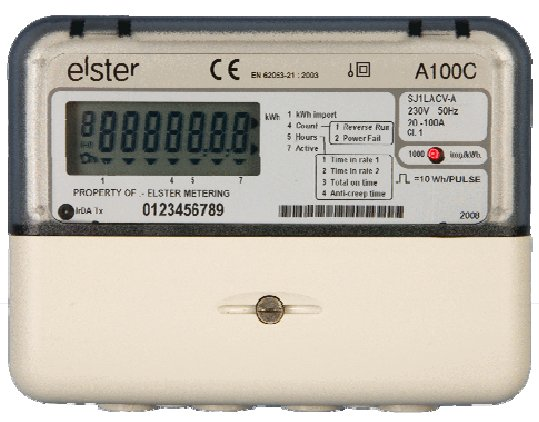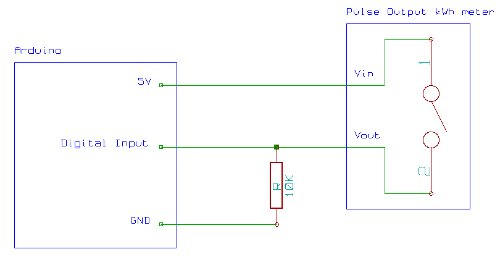# Reading pulses from meters with pulse outputs.. using arduino

A note on what this document covers

The main thing that’s being added here to the wealth of information on the internet about pulse counting is how to count pulses from more than two pulse outputs sources (up to 12 sources) using a continuos sampling and direct port manipulation Arduino sketch as opposed to interrupt driven counting.In the case of counting pulses from one or two pulse output meters it is best to use the interrupt method. There are only two interrupt pins on the Arduino, if you wish to count pulses from more than two sources then please read on!

There is also information here on how to interface with wired / switched pulse outputs.

Interrupt method

Interrupt method sleep

## Introduction

Many meters have pulse outputs, including electricity meters: single phase, 3-phase, import, export.. Gas meters, Water flow meters etc

The pulse output may be a flashing LED or a switching relay (usually solid state) or both.

In the case of an electricity meter a pulse output corresponds to a certain amount of energy passing through the meter (Kwhr/Wh). For single-phase domestic electricity meters (eg. Elster A100c) each pulse usually corresponds to 1 Wh (1000 pulses per kwh).  For the case of higher power meters (often three-phase) each pulse corresponds to a greater amount of energy eg. 2whr per pulse or even 10whr per pulse.

A100C BS Single Phase Meter

Figure 1 illustrates a pulse output. The pulse width T_high varies depending on the pulse output meter. Some pulse output meters allow T_high to be set. T_high remains constant during operation. For the A100c pulse output meter T_high is 50ms. The time between the pulses T_low  is what indicates the power being measured by the meter.Calculating Energy
For the A100c meter each pulse represents a 1000th of a KWhr of 1Whr of energy passing through the meter.

Calculating Power
3600 seconds per hour = 3600J per pulse ie. 1 Whr= 3600J
therefore instantaneous power P=3600/T where T is the time between the falling edge of each pulse.

For more detail: Reading pulses from meters with pulse outputs..

#### This Post / Project can also be found using search terms:

• read pulses electricity esp8266 logger
• pulsemeter arduino
• arduino counting pulses
• semiconductor pulsed output kwhr meter